# Related Distinct CountThe Related Distinct Count pattern allows you to apply the distinct count calculation to any column in any table in the data model. Instead of just counting the number of distinct count values in the entire table using only the DISTINCTCOUNT function, the pattern filters only those values related to events filtered in another table.

For example, you can calculate the number of distinct products bought in a particular period, but you can also count the number of distinct categories bought in a period, even if the category is in a column of a table different from the one containing the sales, which defines who bought what and when.

## Basic Pattern Example

Suppose you want to provide a distinct count measure over a column in a table, considering only the rows that are referenced by a related table. The data model must contain at least one relationship between two tables, and the column you want to count should be in the lookup table (on the “one” side of the one-to-many relationship). In Figure 1, Products is the lookup table and Sales is the related table.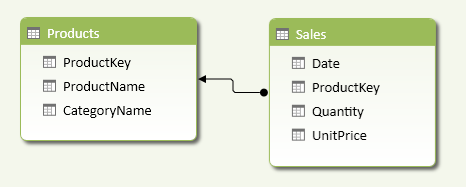Figure 1 The target of the distinct count calculation is the columns in the Products table (the lookup table).

Considering the Products and Sales tables shown in Figure 2, you want to count how many distinct products and categories have been sold every day.

As shown in Figure 3, you compute the number of distinct products and categories sold for every day and as a grand total for the entire table.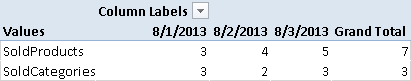Figure 3 The SoldProducts and SoldCategories measures compute the distinct count for sales made in every day and in the entire Sales table.

You define the SoldProducts and SoldCategories measures as follows:

```SoldProducts := DISTINCTCOUNT ( Sales[ProductKey] )

SoldCategories :=
CALCULATE (
DISTINCTCOUNT ( Products[CategoryName] ),
Sales
)
```

Only the SoldCategories measure uses the Related Distinct Count pattern. The SoldProduct measure uses a simple DISTINCTCOUNT function, because it uses the ProductKey column in the Sales table, which is the same table that contains the events you want to use as filter on the distinct count calculation.

The important part of the pattern is that the DISTINCTCOUNT function is included within a CALCULATE function that receives the related table as a filter argument. In this way, any filter active on Sales (the related table) propagates to the lookup table, so the DISTINCTCOUNT function only considers those products used by rows filtered in the Sales table.

In order to understand the importance of the pattern, compare the previous results with the ListProducts and ListCategories measures, defined as follows:

```ListProducts := DISTINCTCOUNT ( Products[ProductKey] )

ListCategories := DISTINCTCOUNT ( Products[CategoryName] )
```

You can see their results in Figure 4: the total for each day and the grand total always calculate to the same value, because the filters active on the Sales table do not propagate to the Products table.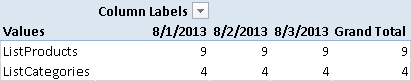Figure 4 The ListProducts and ListCategories measures ignore filters on columns of related tables.

## Use cases

You can use the Related Distinct Count pattern whenever you want to calculate the number of distinct occurrences in a column of a table that has a relationship with a table containing events you are filtering from other tables. This is very common when you have a relational model such as a star schema or a snowflake schema.

### Distinct Count on Dimension Attribute

When you extract data from a data mart modeled as a star schema or snowflake schema, the tables containing qualitative information are called dimensions. Different types of dimensions differ in the way they handle changes of attributes over time. Regardless of dimension type, you can always apply the Related Distinct Count pattern to slowly changing dimensions in star and snowflake schemas, adapting the pattern to your data model.

## Complete Pattern

Whenever you have tables with relationships between them, you can apply the Related Distinct Count pattern if the calculation is on a column of a lookup table. In order to filter the distinct count calculation on a lookup table to consider only the values connected to rows filtered in the related table, you write the DISTINCTCOUNT expression in a CALCULATE statement that contains the related table in its filter argument.

The diagram in Figure 5 shows a data model where you can filter the Sales table by date, product, subcategory, and category. The columns in the Products table represent a star schema, whereas those in the Subcategories table represent a snowflake schema.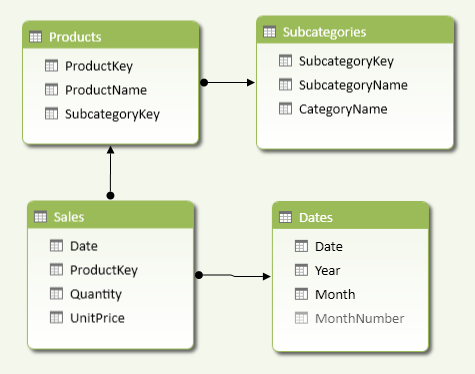Figure 5 The Sales table is the fact table of a snowflake schema, having two levels of relationships connecting to the Subcategories table.

Regardless of the structure represented, you always use the same DAX pattern to obtain the distinct count of categories or subcategories. You can apply the same CALCULATE pattern to categories and subcategories, as shown below.

```SoldSubcategories :=
CALCULATE (
DISTINCTCOUNT ( Products[SubcategoryKey] ),
Sales
)

SoldCategories :=
CALCULATE (
DISTINCTCOUNT( Subcategories[CategoryName] ),
Sales
)
```

The filter argument of the CALCULATE function contains the Sales table, which extends its filter to all its lookup tables, including Products, Subcategories, and Dates. All lookup tables are part of the extended filter, regardless of the number of relationships traversed. By passing the Sales table (which is the equivalent of a fact table in a star or snowflake schema), you apply this extended filter to the existing filter context. For example, you might have a filter on a CategoryName and you want to calculate how many distinct subcategories have been sold.

## More Pattern Examples

In this section, you see a few examples of the Related Distinct Count pattern.

### Simple Distinct Count

You do not need to use the Related Distinct Count pattern if you want to calculate the distinct count on a column in a table containing events that you already filter. You only need the Related Distinct Count pattern when you apply the calculation to a column in a lookup table, not when you apply it to a column in the related table. For example, no column of the Sales table needs the Related Distinct Count pattern, because it is already implicit in the current filter context.

```SoldProducts := DISTINCTCOUNT ( Sales[ProductKey] )

DaysWithSales := DISTINCTCOUNT ( Sales[Date] )
```

If you apply the Related Distinct Count pattern, you will obtain the same results. However, it is unnecessary to apply the pattern when the table specified in the filter argument of the CALCULATE function is the same table to which the column passed to the DISTINCTCOUNT function belongs. For example, in the definitions below, the Sales table has the columns used as an argument for DISTINCTCOUNT as well as being the second argument for CALCULATE.

```SoldProducts :=
CALCULATE (
DISTINCTCOUNT ( Sales[ProductKey] ),
Sales
)

DaysWithSales :=
CALCULATE (
DISTINCTCOUNT ( Sales[Date] ),
Sales
)
```

Thus, there is no need to use the CALCULATE function for the Related Distinct Count pattern if you write a simple distinct count calculation.

### Distinct Count on Attribute in a Star Schema

Use the Related Distinct Count pattern whenever you apply a distinct count on an attribute that is included in a table that has a direct relationship to the table that contains events that you want to filter.

For example, Products and Dates tables in Figure 5 have a relationship with the Sales table, which contains the transactions executed. If you want to calculate the number of months in which you had at least one sale for a given product, or how many product subcategories had at least one sale in a given period, you have to include the Sales table in the filter argument of the CALCULATE function, as shown in the following examples:

```MonthsWithSales :=
CALCULATE (
DISTINCTCOUNT ( Dates[MonthNumber] ),
Sales
)

SoldSubcategories :=
CALCULATE (
DISTINCTCOUNT ( Products[SubcategoryKey] ),
Sales
)
```

The filter argument used in CALCULATE is always a related table from the point of view of the column you specify as the argument of DISTINCTCOUNT.

### Distinct Count on Attribute in a Snowflake Schema

Use the Related Distinct Count pattern whenever you apply a distinct count on an attribute that is included in a table that has more than one relationship to the table that contains events that you want to consider as filter.

For example, the Subcategory table in Figure 5 has a relationship to the Products table, which has a relationship to the Sales table. If you want to calculate how many product categories had at least one sale in a given period, you have to include the Sales table in the filter argument of the CALCULATE function, as shown in the following example:

```SoldCategories :=
CALCULATE (
DISTINCTCOUNT ( Subcategories[CategoryName] ),
Sales
)
```

The filter argument used in CALCULATE is always a related table from the point of view of the column you specify as an argument of DISTINCTCOUNT. Such a related table usually only has lookup tables and does not have cascading related tables. For example, it would be an error to write the following expression:

```WrongCalculation :=
CALCULATE (
DISTINCTCOUNT ( Subcategories[CategoryName] ),
Products
)
```

The WrongCalculation above calculates how many distinct categories are included in the current selection of Products, regardless of the current selection of Sales. In Figure 6, you can see that WrongCalculation always returns the same number for any date, because the date filters the Sales table and not the Products table. The Sales table must be included as a filter in the CALCULATE arguments in order to apply a filter to the lookup tables (including Products and Subcategories).Figure 6 The WrongCalculation always returns the same number regardless of the day in the rows.

### Distinct Count on Slowly Changing Dimension Type 2

A slowly changing dimension is a table that contains several rows for the same entity, because you have different versions of it. In Figure 7, you see on the left a Customers table where a customer (Sophia Turner) has two rows, describing two different cities in which she lived; on the right you see that Sophia bought products when she lived in New York (CustomerKey is 2) and then when she lived in Boston (CustomerKey is 3).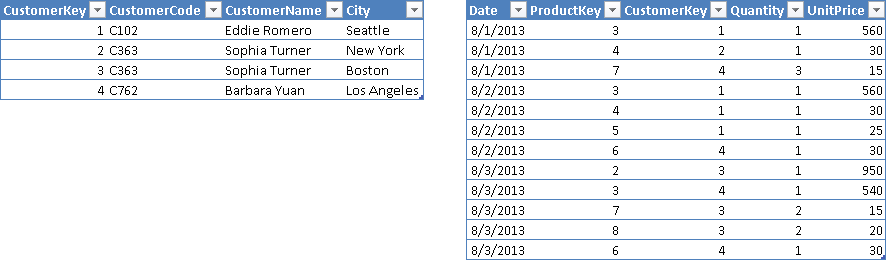Figure 7 The Customers table contains one row for each version of the customer. The CustomerKey column in the Sales table shows the version of the customer who made the transaction.

In Figure 8, you see that the Customers table has a relationship with the Sales table through the CustomerKey column. You should not use such a column in a DISTINCTCOUNT calculation, however.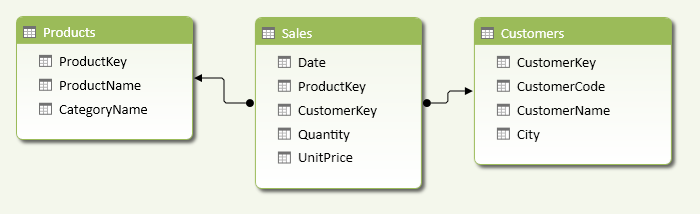Figure 8 The Customers table has a relationship with the Sales table using CustomerKey.

Using the DISTINCTCOUNT function on the CustomerKey column in the Sales table would return the number of distinct versions of customers, and not the number of distinct customers that made a purchase. The following expression is syntactically correct, but returns 4 customers who made transactions in the entire Sales table, whereas the right number should be 3.

`WrongCustomers := DISTINCTCOUNT ( Sales[CustomerKey] )`

In order to calculate the right number of distinct customers, you have to use the CustomerCode column in the Customers table as an argument of the DISTINCTCOUNT function. Since that column is in a lookup table, you have to apply the Related Distinct Count pattern as shown in the following expression:

```UniqueCustomers :=
CALCULATE (
DISTINCTCOUNT ( Customers[CustomerCode] ),
Sales
)
```Enclose code in comments with <PRE></PRE> to preserve indentation.